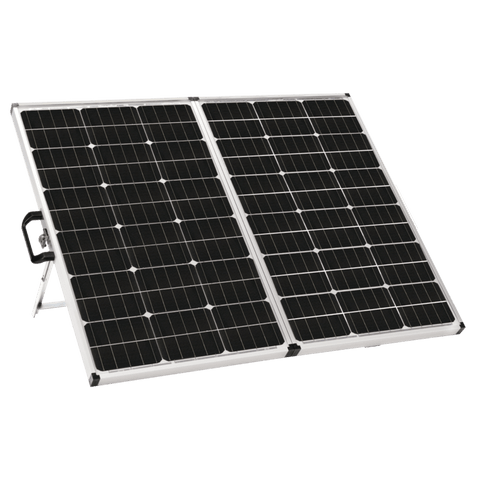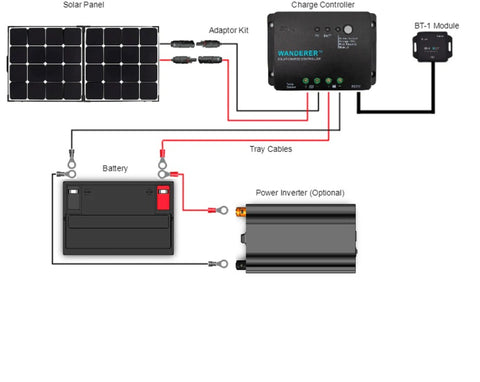Solar Panels Wattage Calculated (How To, Needs, & Sizing) - ShopSolar.com

# Solar Panels Wattage CalculatedThe calculations behind solar panels can be pretty complex if you’re new to the world of solar panels. Of course, we aren’t just referring to calculating a solar panel's wattage/output.

As a solar panel owner, you will be required to calculate the sizing of your solar panel components to the wattage per square meter.

But don’t stress; we got you covered. We will break down the different calculations that need to be performed and the specific factors to consider.

## How to Calculate Solar Panel Output

Before we can start asking questions like how many solar panels you need to power your appliances, we need to do a few calculations.

Calculating a solar panel’s output all comes down to figuring out your solar needs, the efficiency of your solar panel, and the location.

To figure out what your solar needs are, you must write down every appliance that you intend to run off your solar system. This is everything from your computers and phones to your lights and water pumps.### Watt-Hours

It’s important to figure out the power consumption rating level of each appliance.

This is typically found on the appliance itself or included in the manual or box it came in and will be important during the calculations.

Another value to look out for is the output rating of the device, typically listed as watts, volts, or amps.

The wattage is the value you want to look out for, but if it’s not listed, it can be calculated by multiplying the Volts and the amps together, giving you the appliance’s output wattage.

If you can’t see any values on your device for some reason, simply locate the model number and do a quick google search. You should be able to find all the information you need.

Along with the wattage, you should write down how much time in hours you spend a day using the appliances.

For example, if you use a cell phone for only 30 minutes per day, you would write down 0.5 hours.

For the calculation, you want to take the watts and multiply them by the hours to get the watt-hours per day.

You simply repeat this calculation for every appliance you have listed, and voila, you will have the appliance’s corresponding watt-hours.

You want to add up all the watt-hours, and you will be left with the total amount of watt-hours you would use in a day.

#### Solar Needs and Battery CalculationOnce you have all the values you need, you will need to plug them into a solar calculator that can be found online. These calculations will help you decide how many batteries per solar panel are required.

There are hundreds to choose from, but they all perform the same calculations, so don’t stress out about choosing the correct calculator.

These calculators calculate the size of your battery bank, your solar panel, and your charge controllers, giving you a better perspective on the output.

The battery bank sizing takes your watt-hours per day, how many days your system would run without being exposed to too much sun, and the effective capacity of your battery due to low temperatures.

The calculations take certain drops and inconsistencies into consideration hence why it’s easier to allow the calculator to do the calculations.

You should be left with the number of amp-hours needed for your battery bank, which is important to those wanting to invest in solar generators as they categorize the readings into amp hours.

The amp hours needed for your battery is how you would size your battery bank.

#### Solar Panel Output and Sizing

Next, we have how to calculate solar panel and charge controller sizing.

You will be required to enter your home state and city and manually enter the average sun-hours for that location.

Before solar panels are sent out onto the market, they are exposed to 1,000 watts of sunlight per square meter.We refer to this as peak sun hour, and the manufacturers concluded that the amount of daily peak sun hours would differ worldwide.

Solar panels produce more electricity in places that have greater peak sun hours.

If you aren’t too sure about the sun hours in a specific state, there are plenty of maps showing the world solar insolation values with different amounts of sunlight per day based on your location.

Once these two values are entered, the calculator calculates the number of watts you need based on your power consumption.

This allows you to determine the sizing of your solar panels based on their output.

The different outputs on a solar panel, such as 150W or 200W, can be entered, and the calculator will tell you how many solar panels you would need to give you the total wattage.

This number will always be more than the number of watts you need but it overcompensates the number of watts you need.

This is because it calculates a margin that’s closer to the amount of power you need.

#### Solar Panel Charge Controller

The final calculation performed by the solar calculator is the solar charge controller sizing.

It will return a value in amps of saying that you will need a charge controller that can handle that specific value that’s returned. This current travels between the solar panels and batteries or inverters.

Essentially, the solar panel charge controller or regulator is hooked up between the battery and the solar panel. It regulates the battery charging process by preventing any chances of it overcharging.

They’re essential to those wanting to have a solar panel system. It keeps your batteries or solar generators fed and safe to last for years to come.

Now that we’ve gone over all the calculations, we can look at the solar panel’s wattage per square meter.## Solar Panel Wattage Per Square Meter

There are a few factors to consider to determine a solar panel’s wattage per square meter.

First is the number of solar panels on your roof or in your array. Next, you need to consider things like a chimney or dormer window as this restricts your surface area.

The ideal angle of a roof is around 35 degrees as the solar panels are the most efficient and have the highest performance per square meter.

Having a roof slope of around 20 degrees will cause a drop in your solar panels' performance by at least 10%.

When your roof faces south, the solar panels tend to be the most effective and have a higher performance. However, the yield per square meter decreases by 10% on roofs that face a southeast or southwest direction.

The next factor is the power of the panel measured in watt peak. If your solar panel generates around 20,000W per year, the average watt peak will be around 275W. Generally, the more expensive a solar panel is, the higher its peak watts.

The type of solar panel you choose also influences the solar panel’s wattage per square meter. For example, suppose you opt for one of Shop Solar Kit's monocrystalline portable solar panels over a polycrystalline one. In that case, the former tends to have a greater performance wattage per square meter.

There are various calculators available online to get the solar panel wattage per square meter, so don’t stress about needing to write down numbers and subtract or divide them.

Knowing your solar panel wattage per square meter will also allow you to figure out how many solar panels per acre you will need.

But now that we covered watts what about the same concept in kilowatt-hours per square meter?

### Solar Panels kWh Per Square MeterA kilowatt-hour is a measure of energy consumption where an appliance uses 1,000 watts for an hour. Your electric bill typically shows the number of kilowatt-hours you would use in a month.

If you want to get your daily usage, take your monthly usage, and divide it by the number of days in that month. The national average is typically around 30-kilowatt hours per day.

You take the value of the kilowatt-hours per day and divide it by the daily hours of sun. This will give you the kilowatts needed.

A modern solar panel will typically produce between 250 and 270 watts of peak pour during controlled conditions.

They convert the DC power to usable AC, but there are losses in converting the energy from the sun, leading to only about 80% of the DC power being converted.

You will take the kilowatts needed value and divide it by 0.8 to get the required kilowatts in DC. This value will then be divided by the solar panel’s rating and will give you the number of solar panels you will need to compensate for your power requirements.

Different types of solar panels have different yields per square meter.

The average household will need a minimum of 3,500 kWh of electricity, so you would need approximately 28 square meters of solar panels to meet that requirement, assuming you opt for solar panels ranging from 130 to 200 kWh per year.

#### The Average HomeIf you wanted to know how much solar panels cost for a 1,500-square-foot house, that’s where these calculations come into play.

A typical 1500-square-foot house will use around a 6-kilowatt-hour solar system.

Including everything from installation to the cost of the individual solar panels can run you up to \$18000, so it’s not the cheapest at first glance.

However, solar panels are investments that you look at in the long run.

Not only does it save you from paying a hefty electric bill at the end of the month, but you will be making use of a highly sustainable and renewable source of energy.

Did You Find Our Blog Helpful? Then Consider Checking: